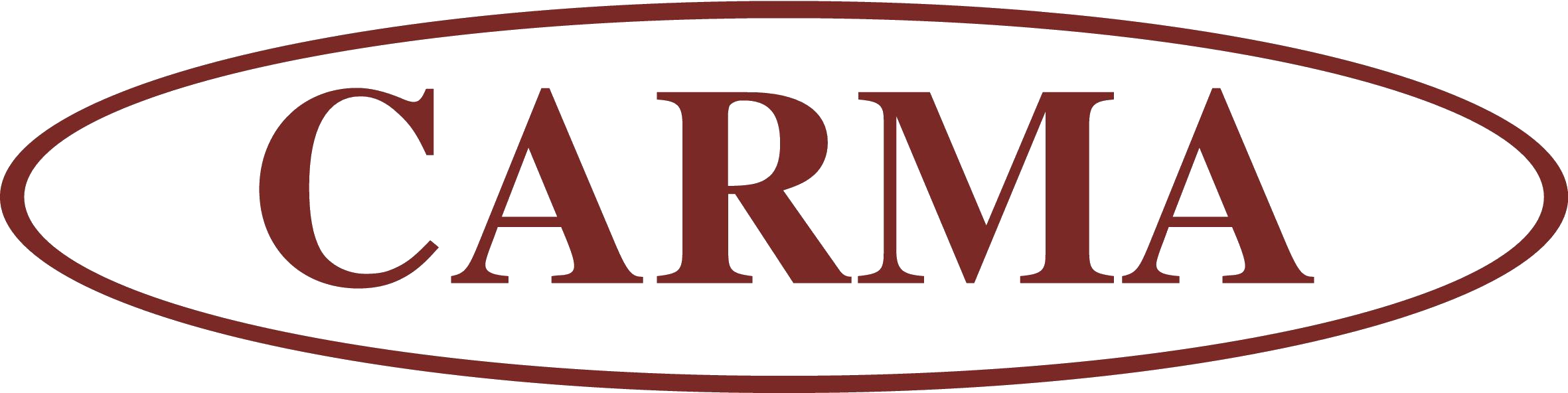# CARMA Discrete Mathematics Seminar

## Thursday, 5th Mar 2015

V106, Mathematics Building

# Dr Yuqing Lin

(School of Electrical Engineering and Computer Science, The University of Newcastle)

# The number of maximal state circles of plane graphs

It is well known that there is a one-to-one correspondence between signed plane graphs and link diagrams via the medial construction. The relationship was once used in knot tabulations in the early time of study in knot theory. Indeed, it provides a method of studying links using graphs. Let $G$ be a plane graph. Let $D(G)$ be the alternating link diagram corresponding to the (positive) $G$ obtained from the medial construction. A state $S$ of $D$ is a labeling each crossing of $D$ by either $A$ or $B$. Making the corresponding split for each crossing gives a number of disjoint embedded closed circles, called state circles. We call a state which possesses maximum number of state circles a maximum state. The maximum state is closely related to the genus of the corresponding link, thus has been studied. In this talk, we will discuss some of the recent progress we have made on this topic.